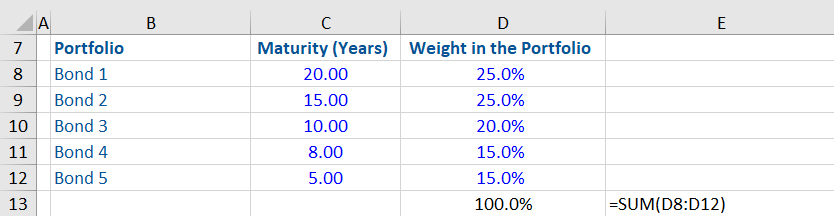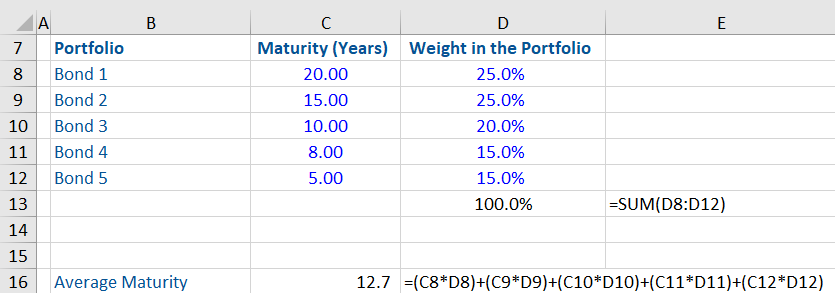## What is “Average Maturity”?

The average maturity is the weighted average of maturities of various bonds held in a portfolio. A bond’s maturity date is a specified date in the future on which the principal amount (the amount borrowed) becomes due for repayment. A portfolio can have multiple bonds, and each bond may have a different maturity period. Average maturity helps in calculating the average maturity period for all the bonds in a portfolio. Some bonds may be larger than others, in which case they are given greater “weight” in the average maturity calculation.

The maturity of most corporate bonds, in practice, ranges between 1 – 30 years. They are often grouped into time periods, being short-term (less than 5 years), medium-term (5 to 12 years) and long-term maturities (over 12 years). However, some companies and governments have issued 100-year bonds too.

## Key Learning Points

• Average maturity shows the weighted average of maturity periods of different bonds in a portfolio. The weights represent the percentage of each bond’s value in the overall portfolio
• Bond prices have an inverse relationship with interest rates. When interest rates fall, the prices of fixed-rate bonds rise, and vice versa.
• Average maturity helps in showing the maturity of the overall portfolio based on the maturities of individual bonds. Portfolios with longer average maturities are more sensitive to interest rate risks when compared to portfolios with shorter average maturities.
• Longer-term bonds carry higher interest risks when compared to short-term bonds.

## Average Maturity and Interest Rate Risk

Average maturity helps in understanding the sensitivity of a portfolio to interest rate changes i.e. comprehending the relationship between maturity of the bonds in the portfolio and interest rate risk.

Basically, a high average maturity indicates that the portfolio has bonds with longer maturities, whereas a lower average maturity indicates bonds with shorter maturities. This matters because a portfolio of bonds with a higher average maturity is more vulnerable to interest rate risks than a portfolio with a shorter average maturity.

It’s important to note two key points.

1. First, investors are concerned about the sensitivity of bond prices to changes in interest rates, as they are exposed to losses if the bond prices drop. This makes bonds risky even if the coupon and principal payments are guaranteed.
2. Second, bond prices and interest rates are inversely related i.e. when interest rates go down, the price of fixed-rate bonds increase and vice versa.

To show how average maturity and interest rate risk relate to each other, suppose a bond is issued with a coupon rate of 4.0%. Thereafter, if market interest rates rise to 5.0%, then who would purchase a 4.0% coupon bond at par or face value. Therefore, the bond price must drop until the expected return rises to the competitive level of 5.0%. Conversely, the opposite happens when market interest rates drop i.e. existing bonds become more attractive and their prices rise.

Therefore, long-term bonds carry a higher interest rate risk i.e. there is more opportunity for interest rate changes to affect a long-term bond than short-term bonds.

This relationship is described using the time value of money. If market interest rates rise, the bond is less valuable because its cash flows are discounted at a higher rate, and the higher discount rate is applied to more distant cash flows, meaning the present value of cash flows (i.e. the bond price) will be that much lower.

Therefore, when interest rates rise (for example, from 4.0% to 5.0%), the percentage fall in the value of the longest term bond (e.g. a 20-year bond) will be significantly more than the fall in the value of a 1-year or 5-year bond.

## Example: Average Maturity

Given below is a workout, in which the average maturity of a bond portfolio is calculated from the information given below.From the table above it can be seen that the 5 bonds in this portfolio have different maturities and carry different weights in the overall portfolio. Bonds with longer maturities have a higher weight in this portfolio e.g. the 20 year bond has a 25.0% weight.

The average maturity is calculated by multiplying each bond’s maturity with its weight and thereafter adding the products.The average maturity of this portfolio is 12.7 years.